Constructing a triangle using RHS congruency criteria

Practical Geometry
Serial order wise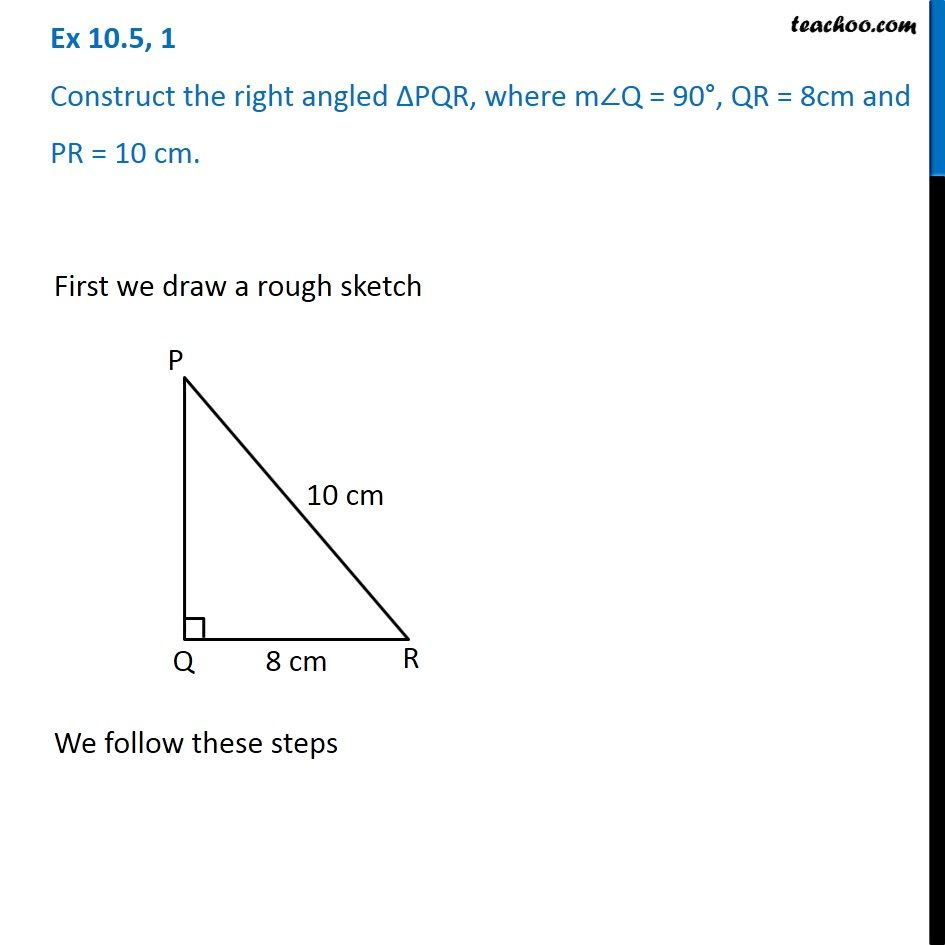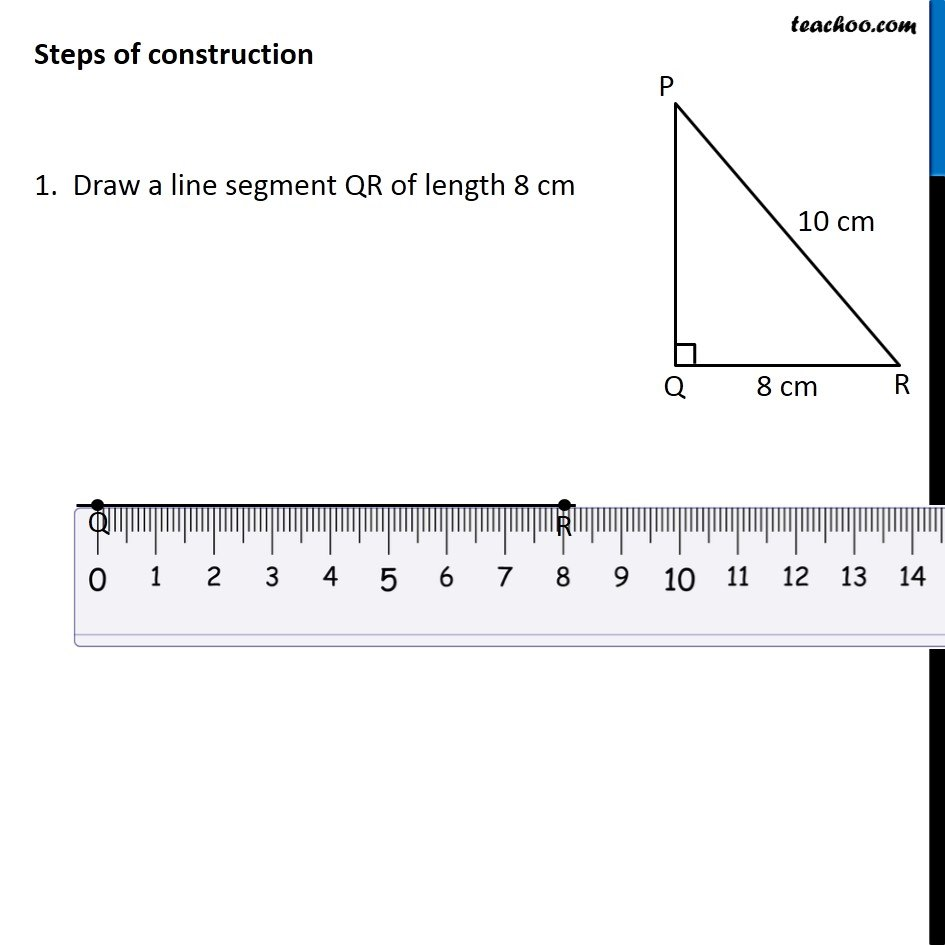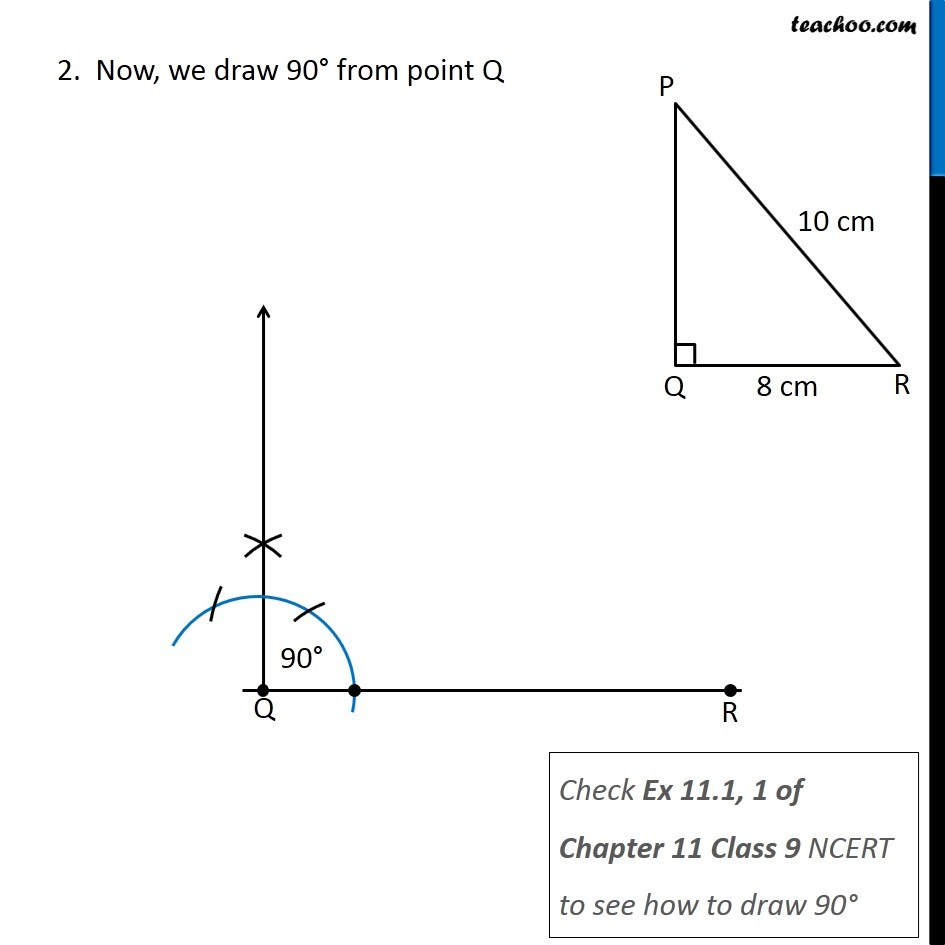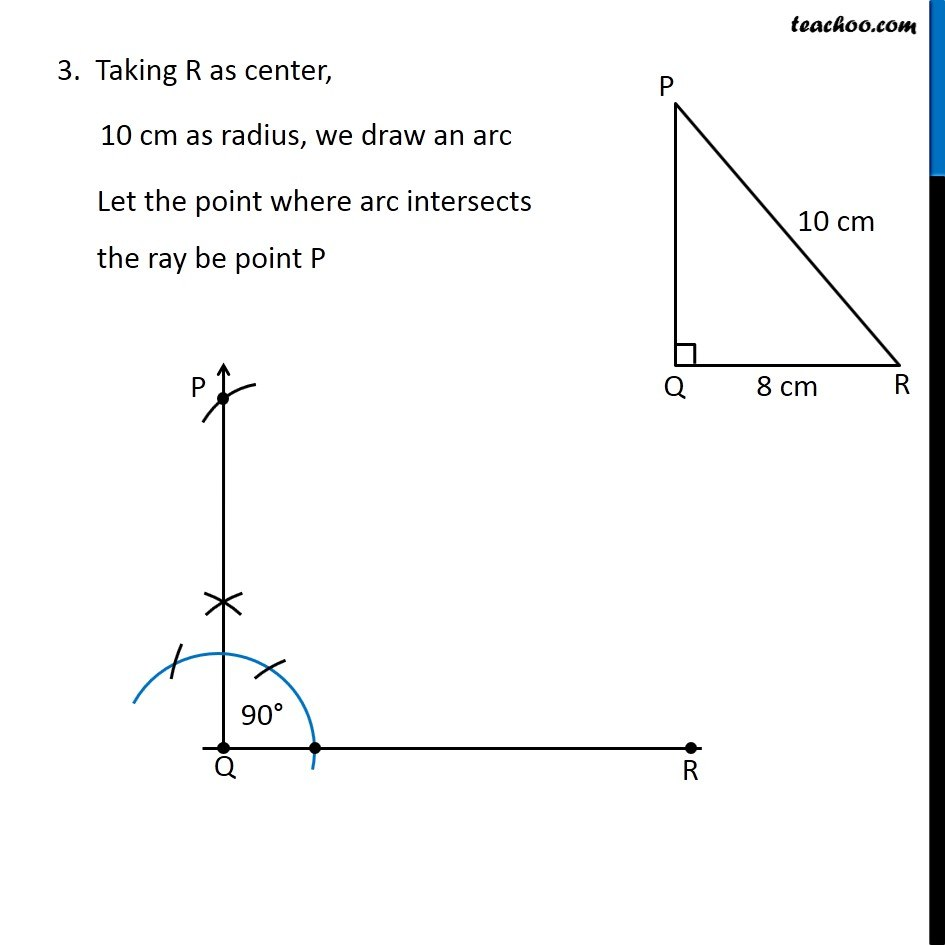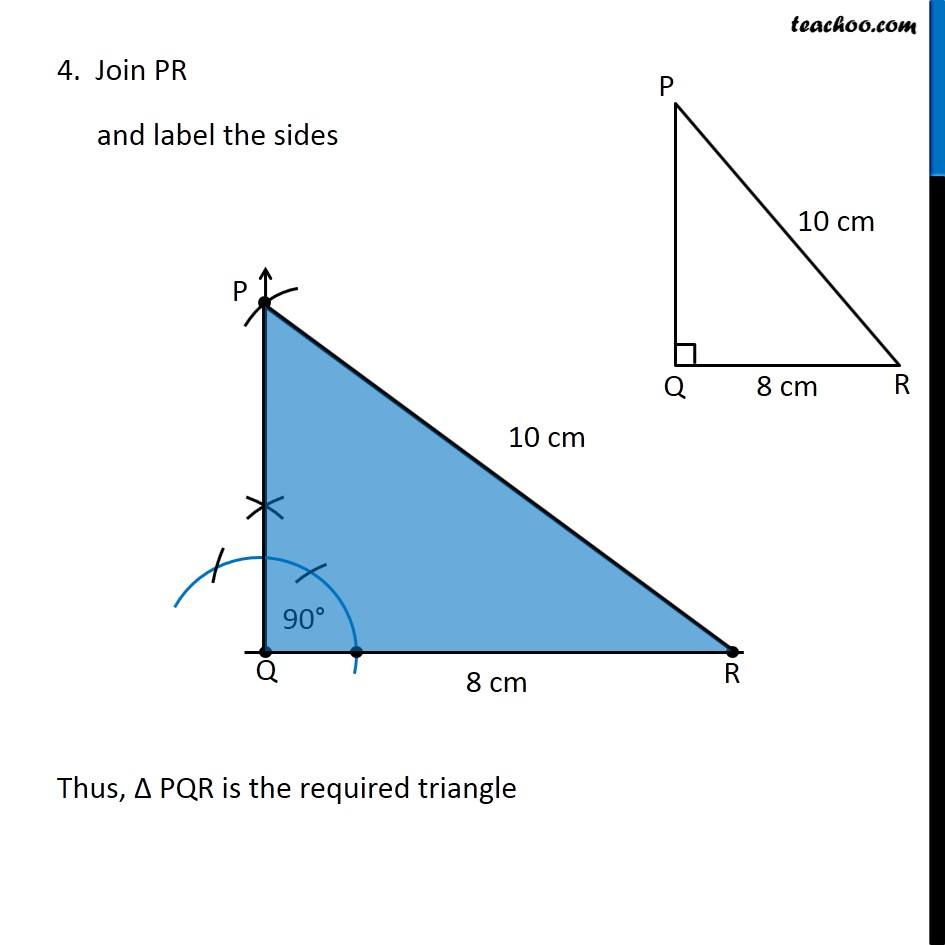Learn in your speed, with individual attention - Teachoo Maths 1-on-1 Class

### Transcript

Question 1 Construct the right angled ∆PQR, where m∠Q = 90°, QR = 8cm and PR = 10 cm. First we draw a rough sketch We follow these steps Steps of construction 1. Draw a line segment QR of length 8 cm 2. Now, we draw 90° from point Q Check Ex 11.1, 1 of Chapter 11 Class 9 NCERT to see how to draw 90° 3. Taking R as center, 10 cm as radius, we draw an arc Let the point where arc intersects the ray be point P 4. Join PR and label the sides Thus, Δ PQR is the required triangle